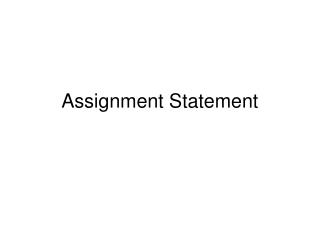DownloadDownload PresentationAssignment Statement

# Assignment Statement

Download Presentation## Assignment Statement

- - - - - - - - - - - - - - - - - - - - - - - - - - - E N D - - - - - - - - - - - - - - - - - - - - - - - - - - -
##### Presentation Transcript

1. Assignment Statement

2. Assignment Statement • Used to perform computations during execution and store the results in main memory • The general form is varible = expression ; • The variable (called Left Hand Side, LHS) must be predeclared • The expression (called Right Hand Side, RHS) can contain: numbers, characters, variables, arithmetic operators, relational operators, logical operators • The assignment statement is executed in two steps: (1) compute the value of the RHS (2) Store the value in main memory in the location indicated by LHS

3. Example int x; float y; char z; x=100; cout<<x; // prints 100 y=10.2; cout<<y; //prints 10.2 z=‘k’; cout<<z; //prints k x=100+1*2; cout<<x; //prints 102 x=!x+3>=2||3&&4; cout<<x; //prints 1

4. Example int x=5, y=6; x=y; y=x; cout<<x<<‘ ’<<y; //prints 6 6 x=y+2; cout<<x<<‘ ’<<y; //prints 8 6 x=x+3; cout<<x<<‘ ’<<y; //prints 11 6

5. Example int x=2; float y=44.73; float z; z=x; //ok cout<<z; //prints 2 x=y; //ok cout<<x; //prints 44

6. Example int x; char y; x=97; y=x; cout<<x<<“ ”<<y; //prints 97 a y=x+2; cout<<y; //prints c y=‘B’; x=y; cout<<x<<“ ”<<y; //prints 66 B x=‘B’+2; cout<<x; //prints 68

7. Assignment Statement Value • The value of an assignment statement is the value of its RHS • Examples int x=7,y,z; cout<<x=8; // prints 8 cout<<x; // prints 8 cout<<x==10; // prints 0 cout<<x; // prints 8 z=y=x; //ok cout<<x<<y<<z; // prints 888

8. Increment and Decrement operations • Increment means an increase in value by 1 • Decrement means a decrease in value by 1 • C provides 4 operation for increment and decrement: • Preincrement (++x): First, increase the value of x by 1. The value of ++x is the new value of x • Postincrement (x++): First, the value of x++ is the old value of x. Then the value of x is increased by 1. • Predecrement (--x): First, decrease the value of x by 1. The value of --x is the new value of x • Postdecrement (x--): First, the value of x-- is the old value of x. Then the value of x is decreased by 1.

9. Example int x=5; cout<<++x; //prints 6 cout<<x; //prints 6 cout<<--x;//prints 5 cout<<x; //prints 5 cout<<x++; //prints 5 cout<<x; //prints 6 cout<<x--; //prints 6 cout<<x; //prints 5

10. Example int x=4, y=5; y=++x; cout<<x<<y; //prints 55 y=--x; cout<<x<<y; //prints 44 y=x++; cout<<x<<y; //prints 54 y=x--; cout<<x<<y; //prints 45

11. Example int x=5, y=8, z; z=++x*y--; cout<<x<<“ ”<<y<<“ ”<<z; //prints 6 7 48 Details: ++x*y-- = 6*y-- = (x value becomes 6) 6*8= (y value becomes 7) 48

12. Assignment Operators • The operator += adds the value of the RHS to the LHS variable • The operator -= subtracts the value of the RHS from the LHS variable • The operator *= multiplies the value of the LHS variable by the value of the RHS • The operator /= divides the value of the LHS variable by the value of the RHS • The operator %= stores the remainder of dividing the current value of the LHS variable by the value of the RHS expression

13. Examples x+=20; // x=x+20; a-=b*c; // a=a-(b*c); c*=(a+b)/c; // c=c*((a+b)/c) y/=x; // y=y/x; y%=h-v; // y=y%(h-v);

14. Priorities 1. ( ) 2. ++ Associate from right to left -- Associate from right to left ! Associate from right to left (type) Associate from right to left unary - Associate from right to left 3. * Associate from left to right / Associate from left to right % Associate from left to right 4. + Associate from left to right - Associate from left to right

15. Priorities Cont. 5. < Associate from left to right <= Associate from left to right > Associate from left to right >= Associate from left to right 6. == Associate from left to right != Associate from left to right 7. && Associate from left to right 8. || Associate from left to right 9. = Associate right to left += Associate right to left -= Associate right to left %= Associate right to left /= Associate right to left *= Associate right to left

16. Example: Write a program to read 2 real values for a and b and print the value of the expression. (a+b)2 |a2 – ab| #include <iostream.h> #include <math.h> void main(){ double a, b, numen, denom; cout<<“Input 2 values:”; cin>>a>>b; numen=pow(a+b,2); denom=abs(a*a-a*b); cout<<“expression value=”<<numen/denom; cout<<endl; }

17. Example: Let f(x)=x2-2x+3. Write a program to read two real values a and b and compute f(a)-f(b). #include <iostream.h> void main(){ double a, b,y1,y2; cout<<“Input 2 values:”; cin>>a>>b; y1=a*a-2*a+3; y2=b*b-2*b+3; cout<<“f(”<<a<<“)-f(”<<b<<”)=”<<y1-y2; cout<<endl; }

18. Example: write a program to read a 3-digits number and print its sum of digits. #include <iostream.h> void main(){ int num, d1, d2, d3; cout<<“Input an integer:”; cin>>num; d1=num%10; num/=10; d2=num%10; num/=10; d3=num; cout<<“SUM=”<<d1+d2+d3; cout<<endl; }

19. Exercizes • Write a program to read an integer of 3 digits and print each digit on a separate line • Write a program to read an integer of 3 digits and print it in reverse order